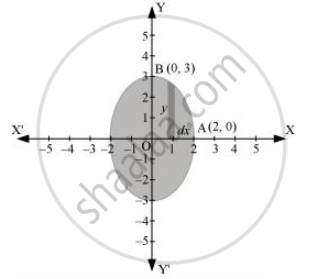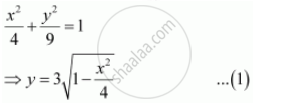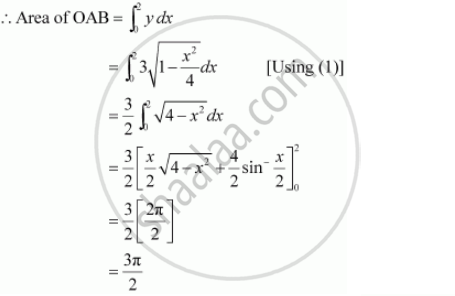# Find the Area of the Region Bounded by the Ellipse X^2/4 + Y^2/9 = 1 - Mathematics

Find the area of the region bounded by the ellipse x^2/4 + y^2/9 = 1

#### Solution

The given equation of the ellipse can be represented asIt can be observed that the ellipse is symmetrical about x-axis and y-axis.

∴ Area bounded by ellipse = 4 × Area OABTherefore, area bounded by the ellipse =  4 xx (3pi)/2 = 6pi units

Concept: Area Under Simple Curves
Is there an error in this question or solution?
Chapter 8: Application of Integrals - Exercise 8.1 [Page 366]

#### APPEARS IN

NCERT Class 12 Maths
Chapter 8 Application of Integrals
Exercise 8.1 | Q 5 | Page 366
Share